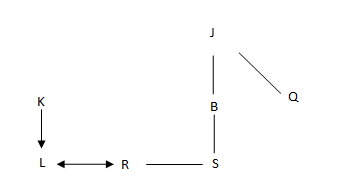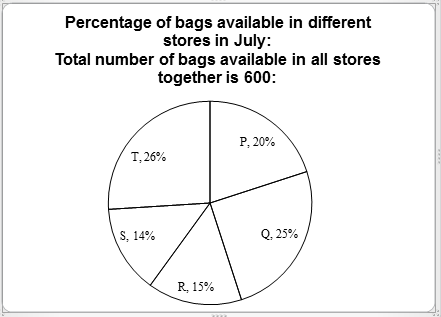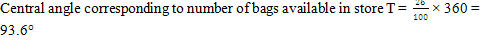# Nokia Reasoning Question

DIRECTIONS for question 1 to 2: Study the following question and mark the best possible option
#WU7*C3&K©Fb4E@ZL%V8SQA$N9¥2M65 1. If all the symbols are dropped from the arrangement, which of the following will be the tenth from the left end of the given arrangement? 1. V 2. 4 3. F 4. L 5. Z Answer: Option E. # W U 7 * C 3 & K © F b 4 E @ Z L % V 8 S Q A$ N 9 ¥ 2 M 6 5
If all the symbols are dropped from the arrangement, then the series is:
W U 7  C 3 K F  4 E Z L  V 8 S Q A  N 9 2 M 6 5
Z will be the tenth from the left end of the given arrangement.
2. Four of the following five are alike in a certain way based on their positions in the given arrangement and so form a group. Which is the one that does not belong to that group?
1. Z%E
2.  L@V
4. 4F@
5. .\$Q9
Starting from option 2, L is placed in the centre. On it’s both sides, one space each will be followed by @ to left and V towards its right. Therefore, L@V. Same as in the case of &C©, C is placed in the centre. On it’s both sides’ one space each will be followed by '&' to left and © towards its right. This pattern is followed by all other option except Z%E.
Z%E is not belonging to that group.
DIRECTIONS for question 3 to 5:
L is the only child of K. R is married to L. S is the sister of R. S is the only daughter of B. J, the father of B has only two children. Q is the daughter of J.
1. How is J related to S?
1. Grandfather
2.  Brother-in-law
3.  Uncle
4.  Cousin
5. Father-in-law
The following arrangement can be drawn based on the given information:J is the grandfather of S.
2. If J has only one daughter, then how is B related to L?
1. Nephew
2. Niece
3. Father-in-law
4.  Brother
5. Mother-in-law
The following arrangement can be drawn based on the given information:If J has only one daughter, this means B is the son of J. Hence, B is the father of R and father-in-law of L.
3. How is Q related to R?
1. Daughter-in-law
2. Grandmother
3. Niece
4. Aunt
5.  Mother-in-law
The following arrangement can be drawn based on the given information:Q is aunt of R.

4. If all the digits of the number 49318 are arranged in ascending order from left to right within the number, then what will be the difference between the second digit from the left and the second digit from the right after the rearrangement?
1. Other than those given as options
2. 5
3. 2
4. 3
5. 4
If all the digits of the number 49318 are arranged in ascending order from left to right within the number, then number become 13489.
After the rearrangement, Second digit from the left is 3 and the second digit from the right is 8, then Difference between them= 8-3=5.
5. In a certain code language,
'pod na joc' means 'very bright boy';
'tam nu pod' means 'the boy comes';
'nu per ton' means 'keep the doll';
'joc ton su' means 'very good doll'.
Which of the following means 'bright' in the same code language?
1. joc
2. Pod
3. Ton
4. Na
5. None of these
In statements (1) and (2), the common code word is 'pod' and the common word is 'boy'.
So, 'pod' stands for 'boy'.
In statements (1) and (4), the common code word is 'joc' and the common word is 'very'.
So, 'joc' stands for 'very'.
So, in (1) 'na' stands for 'bright'.
DIRECTIONS for question 8 to 10: Refer to the pie chart and answer the given question:6. What is the central angle corresponding to number of bags available in store T? (in degrees)
1. 91.2
2. 95.6
3.  93.6
4. 94.2
5. 92.57. What is the difference between the average number of bags available in stores P and R together and the average number of bags available in stores S and T together?
1. 12
2. 22
3. 15
4.  18
5. 20